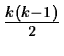Next: Correspondence Analysis Up: Multivariate Analysis Methods Previous: Cluster Analysis

# Discriminant Analysis

Discriminant Analysis may be used for two objectives: either   we want to assess the adequacy of classification, given the group memberships of the objects under study; or we wish to assign objects to one of a number of (known) groups of objects. Discriminant Analysis may thus have a descriptive or a predictive objective.

In both cases, some group assignments must be known before carrying out the Discriminant Analysis. Such group assignments, or labelling, may be arrived at in any way. Hence Discriminant Analysis can be employed as a useful complement to Cluster Analysis (in order to judge the results of the latter) or Principal Components Analysis. Alternatively, in star-galaxy separation, for instance, using digitised images, the analyst may define group (stars, galaxies) membership visually for a conveniently small training set or design set.

Methods implemented in this area are Multiple Discriminant Analysis, Fisher's Linear Discriminant Analysis, and K-Nearest Neighbours Discriminant Analysis.

Multiple Discriminant Analysis
(MDA) is also termed Discriminant       Factor Analysis and Canonical Discriminant Analysis. It adopts a similar perspective to PCA: the rows of the data matrix to be examined constitute points in a multidimensional space, as also do the group mean vectors. Discriminating axes are determined in this space, in such a way that optimal separation of the predefined groups is attained. As with PCA, the problem becomes mathematically the eigenreduction of a real, symmetric matrix. The eigenvalues represent the discriminating power of the associated eigenvectors. The nYgroups lie in a space of dimension at most nY - 1. This will be the number of discriminant axes or factors obtainable in the most common practical case when n > m > nY (where n is the number of rows, and m the number of columns of the input data matrix).

Linear Discriminant Analysis
is the 2-group case of MDA.   It optimally separates two groups, using the Mahalanobis metric or generalized distance.     It also gives the same linear separating decision surface as Bayesian maximum likelihood discrimination in the case of equal class covariance matrices.

K-NNs Discriminant Analysis
: Non-parametric (distribution-free) methods dispense with the need for assumptions regarding the probability density function. They have become very popular especially in the image processing area. The K-NNs method assigns an object of unknown affiliation to the group to which the majority of its K nearest neighbours belongs.

There is no best discrimination method. A few remarks concerning the advantages and disadvantages of the methods studied are as follows.

• Analytical simplicity or computational reasons may lead to initial consideration of linear discriminant analysis or the NN-rule.

• Linear discrimination is the most widely used in practice. Often the 2-group method is used repeatedly for the analysis of pairs of multigroup data (yieldingdecision surfaces for k groups).

• To estimate the parameters required in quadratic discrimination more computation and data is required than in the case of linear discrimination. If there is not a great difference in the group covariance matrices, then the latter will perform as well as quadratic discrimination.

• The k-NN rule is simply defined and implemented, especially if there is insufficient data to adequately define sample means and covariance matrices.

• MDA is most appropriately used for feature selection. As in   the case of PCA, we may want to focus on the variables used in order to investigate the differences between groups; to create synthetic variables which improve the grouping ability of the data; to arrive at a similar objective by discarding irrelevant variables; or to determine the most parsimonious variables for graphical representational purposes.Next: Correspondence Analysis Up: Multivariate Analysis Methods Previous: Cluster Analysis
Petra Nass
1999-06-15